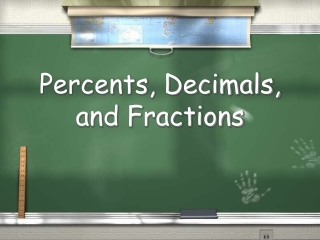DownloadDownload PresentationPercents, Decimals, and Fractions

# Percents, Decimals, and Fractions

Télécharger la présentation## Percents, Decimals, and Fractions

- - - - - - - - - - - - - - - - - - - - - - - - - - - E N D - - - - - - - - - - - - - - - - - - - - - - - - - - -
##### Presentation Transcript

1. Percents, Decimals, and Fractions

2. 1 1 ÷ 2 2 1 2 These all mean the same thing.

3. To change a fraction into a decimal… Divide the numerator (top number) by the denominator (bottom number) 1 2 1 2

4. To change a fraction into a decimal… Add a decimal and zero 1 .0 2 1 2

5. To change a fraction into a decimal… Move the decimal straight up . 1 .0 2 1 2

6. To change a fraction into a decimal… Now just divide . 1 2 1 .0 2

7. To change a fraction into a decimal… And the answer is… 1 0.5 = 2

8. To change a decimal into a fraction… (You have to know place value!) Change 0.8 to a fraction in lowest terms Read it: 0.8 (eight tenths) 8 Write it: 10 4 Reduce it: 5

9. To change a decimal into a fraction… (You have to know place value!) Change 0.09 to a fraction in lowest terms Read it: 0.09 (nine hundredths) 9 Write it: 100 9 Reduce it: (already in lowest terms) 100

10. To change a decimal into a percent… Change 0.09 to a percent Multiply by 100 and add a % sign 9%

11. To change a decimal into a percent… Change 0.09 to a percent 0.09 HINT! HINT! The easiest way to multiply by 100 is to move the decimal 2 places to the right. Answer: 9%

12. To change a decimal into a percent… Change 0.45 to a percent 0.45 HINT! HINT! Answer: 45%

13. Fraction Decimal Percent 1 = = 0.5 50% 2

14. Fraction Decimal Percent 1 = = 0.25 25% 4

15. Fraction Decimal Percent 3 = = 0.75 75% 4

16. Fraction Decimal Percent 3 = = 0.3 30% 10

17. Fraction Decimal Percent 3 = = 0.03 3% 100